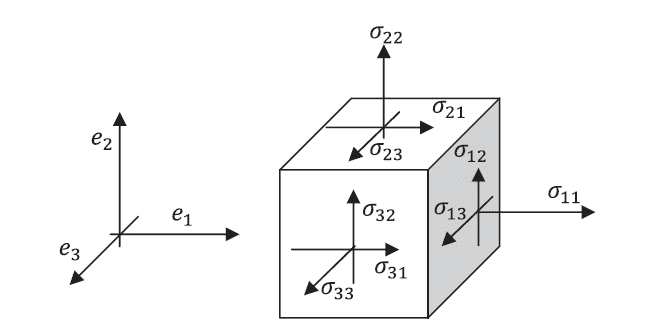# What is a tensor quantity

• B
• vtheone
In summary, a tensor quantity is a type of quantity that can be specified in two directions, and is different from a vector and a scalar quantity. Pressure is a tensor quantity because it has two components, shear stress and pressure.

#### vtheone

What is a tensor quantity and how is it different from vector and scalar qantities? Also why is pressure a tensor quantity?

vtheone said:
What is a tensor quantity and how is it different from vector and scalar qantities? Also why is pressure a tensor quantity?
The best source I could recommend for you on this topic is a book by J.F. Nye "Physical Properties of Crystals and their Representation by Tensors and Matrices". His description of what tensors are all about is extremely clear, and I think you might find it good reading.

•vtheone
Thanks for the advice . I just wanted to know a little more about pressure . I heard some people say pressure is a tensor quantity but someone else told me that it is not pressure but stress that is tensor so I wanted to ask what kind of quantity pressure exactly is? Scalar or vector or tensor or something else.

•vtheone
Let ##X,Y## be finite dimensional vector spaces over the same field, say ##\mathbb{R}##. By ##X'## denote a vector space of linear functions ##f:X\to \mathbb{R}##, and ##Y'## is the same for ##Y##.

Definition. 1) Tensor product ##X\otimes Y## is a vector space that consists of bilinear functions ##f:X'\times Y'\to \mathbb{R}##.
2) tensor product of two elements ##x\in X, \quad y\in Y## is an element of ##X\otimes Y## that is defined as follows ##(x\otimes y)(u,v)=u(x)v(y).##

In the same way one can define a tensor product of any finite number of finite dimensional vector spaces.

Definition. Let ##X## be a finite dimensional vector space. Introduce a space
$$T^p_q(X)=\underbrace{ X\otimes\ldots\otimes X}_{p\, times}\otimes\underbrace{ X'\otimes\ldots\otimes X'}_{q\, times}.$$
Elements of the space ##T^p_q(X)## are called the ##(p,q)-##type tensors of the space ##X##.

•vtheone
To repeat, for the sake of reviewing the material, In a purely technical sense, a (p,q)-tensor is a (p+q)-linear map defined on p covariant variables/arguments and q contravariant ones , so that (1,0)- and (0,1)- tensors are just linear maps, (1,1)-; (2,0)- ; (0,2)- are bilinear maps on a choice of covariant and contravariant variables/arguments, etc. If ## p+q \leq 2## you can represent the tensor as a matrix /quadratic form, otherwise you cannot, at least not in a standard matrix format. EDIT In a Physics sense, AFAIK ( but please double-check) a physical quantity living in (usually ) n-dimensional Euclidean space is a quantity that cannot be fully described with n-or-less data points.

Last edited:
•vtheone
The basic idea behind a tensor is actually a lot simpler than you might imagine, at least if you only want a physical intuition.

When you need to assign a quantity to each direction in the $x$, $y$, and $z$, you get a vector. But many times in physics, you'll have to specify two directions to get one component of a quantity. Whereas a vector $V$'s component may tell you how much of $V$ is in the, say, $x$ direction, a tensor $T$'s component will tell you something like how much of $T$ is in the $y$ direction of the $x$ part of $T$.

Now, for a concrete example - the Cauchy stress tensor (I think this is what you were looking for when you wanted to know why pressure is a tensor):You need two directions to specify one component of this tensor. The first index tells you which plane the stress is acting in; once you know which plane you are in, the second component tells you which direction the stress acts in. These stresses have units of pressure, and the ones acting along the plane actually work to deform, or shear the material.

NOTE: This is only a very crude explanation, meant merely to be physically intuitive, not exhaustive or technically accurate. The real crux of the concept of tensors lies in the fact that these mathematical objects can be 'transformed' in very specific ways. This is, in fact, true of vectors as well (which are actually tensors of rank 1). A vector isn't a vector because it has three components or that it has a direction and magnitude - it is a vectors because it transforms in very specific ways under given transformations; so, for example, if you wanted to know some information about a vector after a specified rotation (say, it's x-component), you could rotate the vector through a rotation transformation, and the resulting mathematical quantity would faithfully give you that information.

I suspect similar things can be done with tensors, though I am not aware of the details. But, even with the Cauchy stress tensor, it seems reasonable that you should be able to get the shear stresses and pressure on an arbitrarily oriented surface by just transforming the tensor (however that may be done).

•vtheone
In a more Physical example, say the stress tensor, it is an object that lives in k dimensions but needs more than k pieces of data to be fully described or for its properties to be fully described. Maybe the Physics people can comment on this, correct if necessary.